# Extract Forest Metrics and Individual Tree Attributes from Aerial Lidar Data

This example shows how to extract forest metrics and individual tree attributes from aerial lidar data.

Forest study and applications increasingly make use of lidar data acquired from airborne laser scanning systems. Point cloud data from high density lidar enables measurement of not only forest metrics, but also attributes of individual trees.

This example uses point cloud data from a LAZ file captured by an airborne lidar system as input. In this example you first extract forest metrics by classifying point cloud data into ground and vegetation points, and then extract individual tree attributes by segmenting vegetation points into individual trees. This figure provides an overview of the process.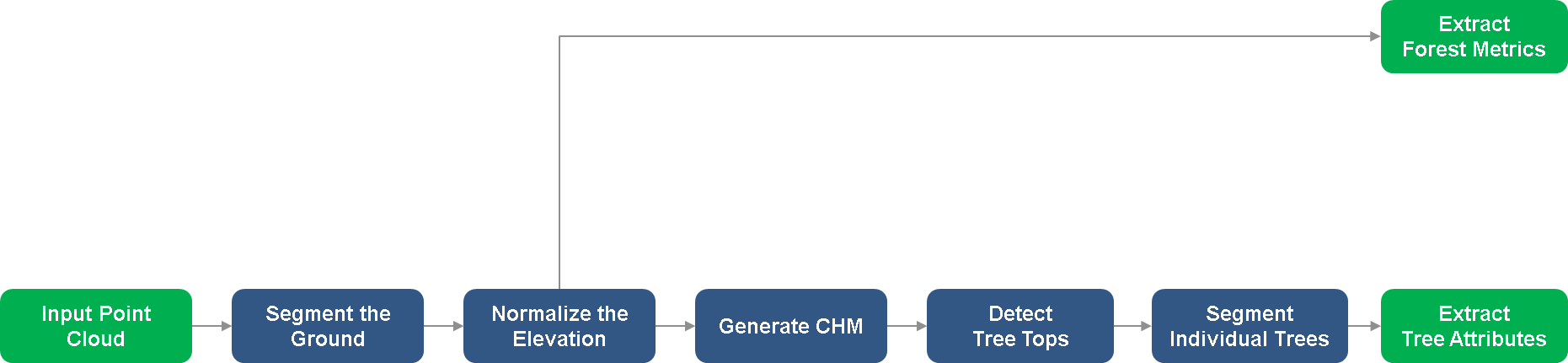Unzip `forestData.zip` to a temporary directory and load the point cloud data from the LAZ file, `forestData.laz`, into the MATLAB® workspace. The data is obtained from the Open Topography Dataset . The point cloud primarily contains ground and vegetation points. Load the point cloud data into the workspace using the `readPointCloud` function of the `lasFileReader` object. Visualize the point cloud using the `pcshow` function.

```dataFolder = fullfile(tempdir,"forestData",filesep); dataFile = dataFolder + "forestData.laz"; % Check whether the folder and data file already exist or not folderExists = exist(dataFolder,'dir'); fileExists = exist(dataFile,'file'); % Create a new folder if it doesn't exist if ~folderExists mkdir(dataFolder); end % Extract aerial data file if it doesn't exist if ~fileExists unzip('forestData.zip',dataFolder); end % Read LAZ data from file lasReader = lasFileReader(dataFile); % Read point cloud along with corresponding scan angle information [ptCloud,pointAttributes] = readPointCloud(lasReader,"Attributes","ScanAngle"); % Visualize the input point cloud figure pcshow(ptCloud.Location) title("Input Point Cloud")```### Segment Ground

Ground segmentation is a preprocessing step to isolate the vegetation data for extracting forest metrics. Segment the data loaded from the LAZ file into ground and nonground points using the `segmentGroundSMRF` function.

```% Segment Ground and extract non-ground and ground points groundPtsIdx = segmentGroundSMRF(ptCloud); nonGroundPtCloud = select(ptCloud,~groundPtsIdx); groundPtCloud = select(ptCloud,groundPtsIdx); % Visualize non-ground and ground points in magenta and green, respectively figure pcshowpair(nonGroundPtCloud,groundPtCloud) title("Segmented Non-Ground and Ground Points")```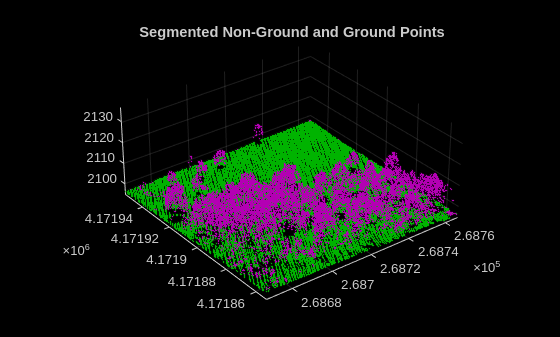### Normalize the Elevation

Use elevation normalization to eliminate the effect of the terrain on your vegetation data. Use points with normalized elevation as input for computing forest metrics and tree attributes. These are the steps for elevation normalization.

1. Eliminate duplicate points along the x- and y-axes, if any, by using the `groupsummary` function.

2. Create an interpolant using the `scatteredInterpolant` object, to estimate ground at each point in the point cloud data.

3. Normalize the elevation of each point by subtracting the interpolated ground elevation from the original elevation.

```groundPoints = groundPtCloud.Location; % Eliminate duplicate points along x- and y-axes [uniqueZ,uniqueXY] = groupsummary(groundPoints(:,3),groundPoints(:,1:2),@mean); uniqueXY = [uniqueXY{:}]; % Create interpolant and use it to estimate ground elevation at each point F = scatteredInterpolant(double(uniqueXY),double(uniqueZ),"natural"); estElevation = F(double(ptCloud.Location(:,1)),double(ptCloud.Location(:,2))); % Normalize elevation by ground normalizedPoints = ptCloud.Location; normalizedPoints(:,3) = normalizedPoints(:,3) - estElevation; % Visualize normalized points figure pcshow(normalizedPoints) title("Point Cloud with Normalized Elevation")```### Extract Forest Metrics

Extract forest metrics from the normalized points using the `helperExtractForestMetrics` helper function, attached to this example as a supporting file. The helper function first divides the point cloud into grids based on the provided `gridSize`, and then calculates the forest metrics. The helper function assumes that all points with a normalized height lower than `cutoffHeight` are ground and the remaining points are vegetation. Compute these forest metrics.

• Canopy Cover (CC) — Canopy cover  is the proportion of the forest covered by the vertical projection of the tree crowns. Calculate it as the ratio of vegetation returns relative to the total number of returns.

• Gap fraction (GF) — Gap fraction  is the probability of a ray of light passing through the canopy without encountering foliage or other plant elements. Calculate it as the ratio of ground returns relative to the total number of returns.

• Leaf area index (LAI) — Leaf area index  is the amount of one-sided leaf area per unit of ground area. The LAI value is calculated using the equation $\mathrm{LAI}=-\frac{\mathrm{cos}\left(\mathrm{ang}\right)*\mathrm{ln}\left(\mathrm{GF}\right)}{\mathit{k}}$, where `ang` is the average scan angle, `GF` is the gap fraction, and `k` is the extinction coefficient, which is closely related to the leaf-angle distribution.

```% Set grid size to 10 meters per pixel and cutOffHeight to 2 meters gridSize = 10; cutOffHeight = 2; leafAngDistribution = 0.5; % Extract forest metrics [canopyCover,gapFraction,leafAreaIndex] = helperExtractForestMetrics(normalizedPoints, ... pointAttributes.ScanAngle,gridSize,cutOffHeight,leafAngDistribution); % Visualize forest metrics hForestMetrics = figure; axCC = subplot(2,2,1,Parent=hForestMetrics); axCC.Position = [0.05 0.51 0.4 0.4]; imagesc(canopyCover,Parent=axCC) title(axCC,"Canopy Cover") axis off colormap(gray) axGF = subplot(2,2,2,Parent=hForestMetrics); axGF.Position = [0.55 0.51 0.4 0.4]; imagesc(gapFraction,'Parent',axGF) title(axGF,"Gap Fraction") axis off colormap(gray) axLAI = subplot(2,2,[3 4],Parent=hForestMetrics); axLAI.Position = [0.3 0.02 0.4 0.4]; imagesc(leafAreaIndex,Parent=axLAI) title(axLAI,"Leaf Area Index") axis off colormap(gray)```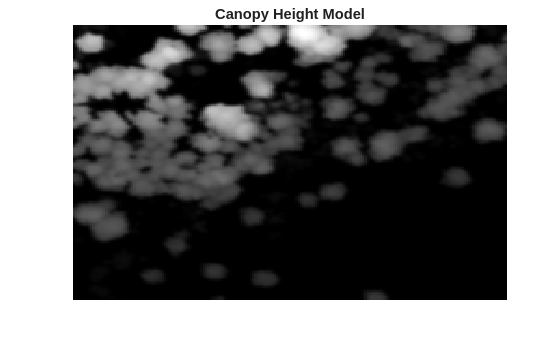### Generate Canopy Height Model (CHM)

Canopy height models (CHMs) are raster representations of the height of trees, buildings, and other structures above the ground topography. Use a CHM as an input for tree detection and segmentation. Generate the CHM from your normalized elevation values using the `pc2dem` function.

```% Set grid size to 0.5 meters per pixel gridRes = 0.5; % Generate CHM canopyModel = pc2dem(pointCloud(normalizedPoints),gridRes,CornerFillMethod="max"); % Clip invalid and negative CHM values to zero canopyModel(isnan(canopyModel) | canopyModel<0) = 0; % Perform gaussian smoothing to remove noise effects H = fspecial("gaussian",[5 5],1); canopyModel = imfilter(canopyModel,H,'replicate','same'); % Visualize CHM figure imagesc(canopyModel) title('Canopy Height Model') axis off colormap(gray)```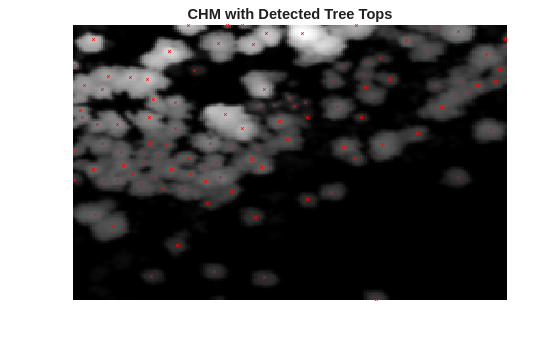### Detect Tree Tops

Detect tree tops using the `helperDetectTreeTops` helper function, attached to this example as a supporting file. The helper function detects tree tops by finding the local maxima within variable window sizes  in a CHM. For tree top detection, the helper function considers only points with a normalized height greater than `minTreeHeight`.

```% Set minTreeHeight to 5 m minTreeHeight = 5; % Detect tree tops [treeTopRowId,treeTopColId] = helperDetectTreeTops(canopyModel,gridRes,minTreeHeight); % Visualize treetops figure imagesc(canopyModel) hold on plot(treeTopColId,treeTopRowId,"rx",MarkerSize=3) title("CHM with Detected Tree Tops") axis off colormap("gray")```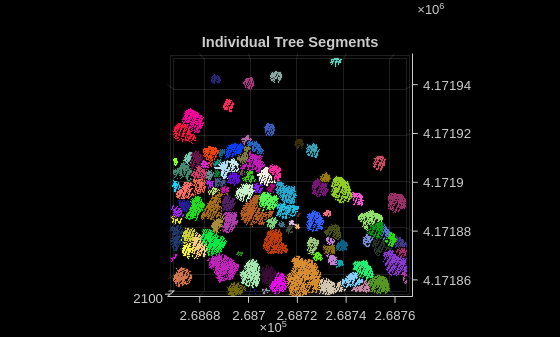### Segment Individual Trees

Segment individual trees using the `helperSegmentTrees` helper function, attached to this example as a supporting file. The helper function utilizes marker-controlled watershed segmentation  to segment individual trees. First, the function creates a binary marker image with tree top locations indicated by a value of `1` . Then, function filters the CHM complement by minima imposition to remove minima that are not tree tops. The function then performs watershed segmentation on the filtered CHM complement to segment individual trees. After segmentation, visualize the individual tree segments.

```% Segment individual trees label2D = helperSegmentTrees(canopyModel,treeTopRowId,treeTopColId,minTreeHeight); % Identify row and column id of each point in label2D and transfer labels % to each point rowId = ceil((ptCloud.Location(:,2) - ptCloud.YLimits(1))/gridRes) + 1; colId = ceil((ptCloud.Location(:,1) - ptCloud.XLimits(1))/gridRes) + 1; ind = sub2ind(size(label2D),rowId,colId); label3D = label2D(ind); % Extract valid labels and corresponding points validSegIds = label3D ~= 0; ptVeg = select(ptCloud,validSegIds); veglabel3D = label3D(validSegIds); % Assign color to each label numColors = max(veglabel3D); colorMap = randi([0 255],numColors,3)/255; labelColors = label2rgb(veglabel3D,colorMap,OutputFormat="triplets"); % Visualize tree segments figure pcshow(ptVeg.Location,labelColors) title("Individual Tree Segments") view(2)```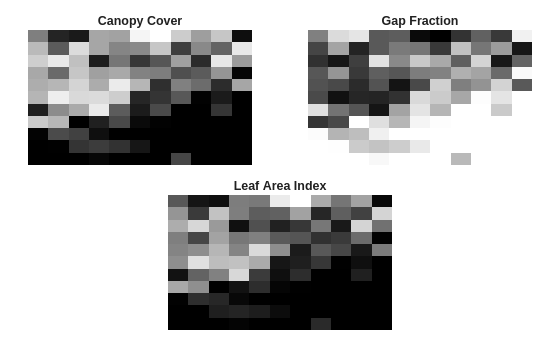### Extract Tree Attributes

Extract individual tree attributes using the `helperExtractTreeMetrics` helper function, attached to this example as a supporting file. First, the function identifies points belonging to individual trees from labels. Then, the function extracts tree attributes such as tree apex location along the x- and y-axes, approximate tree height, tree crown diameter, and area. The helper function returns the attributes as a table, where each row represents the attributes of an individual tree.

```% Extract tree attributes treeMetrics = helperExtractTreeMetrics(normalizedPoints,label3D); % Display first 5 tree segments metrics disp(head(treeMetrics,5));```
``` TreeId NumPoints TreeApexLocX TreeApexLocY TreeHeight CrownDiameter CrownArea ______ _________ ____________ ____________ __________ _____________ _________ 1 388 2.6867e+05 4.1719e+06 29.509 7.5325 44.562 2 22 2.6867e+05 4.1719e+06 21.464 0.99236 0.77344 3 243 2.6867e+05 4.1719e+06 24.201 5.7424 25.898 4 101 2.6867e+05 4.1719e+06 21.927 3.4571 9.3867 5 54 2.6867e+05 4.1719e+06 19.515 3.0407 7.2617 ```

### References

 Thompson, S. Illilouette Creek Basin Lidar Survey, Yosemite Valley, CA 2018. National Center for Airborne Laser Mapping (NCALM). Distributed by OpenTopography. https://doi.org/10.5069/G96M351N. Accessed: 2021-05-14

 Ma, Qin, Yanjun Su, and Qinghua Guo. “Comparison of Canopy Cover Estimations From Airborne LiDAR, Aerial Imagery, and Satellite Imagery.” IEEE Journal of Selected Topics in Applied Earth Observations and Remote Sensing 10, no. 9 (September 2017): 4225–36. https://doi.org/10.1109/JSTARS.2017.2711482.

 Richardson, Jeffrey J., L. Monika Moskal, and Soo-Hyung Kim. "Modeling Approaches to Estimate Effective Leaf Area Index from Aerial Discrete-Return LIDAR." Agricultural and Forest Meteorology 149, no. 6–7 (June 2009): 1152–60. https://doi.org/10.1016/j.agrformet.2009.02.007.

 Pitkänen, J., M. Maltamo, J. Hyyppä, and X. Yu. "Adaptive Methods for Individual Tree Detection on Airborne Laser Based Canopy Height Model." International Archives of Photogrammetry, Remote Sensing and Spatial Information Sciences 36, no. 8 (January 2004): 187–91.

 Chen, Qi, Dennis Baldocchi, Peng Gong, and Maggi Kelly. “Isolating Individual Trees in a Savanna Woodland Using Small Footprint Lidar Data.” Photogrammetric Engineering & Remote Sensing 72, no. 8 (August 1, 2006): 923–32. https://doi.org/10.14358/PERS.72.8.923.

## SupportGet trial now